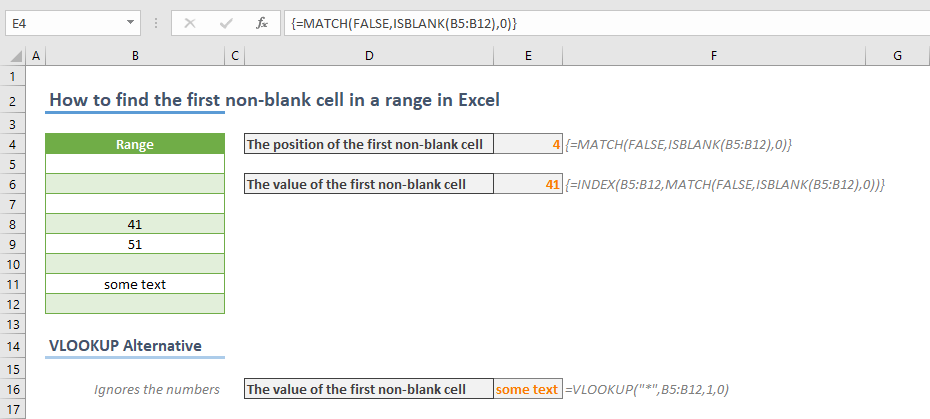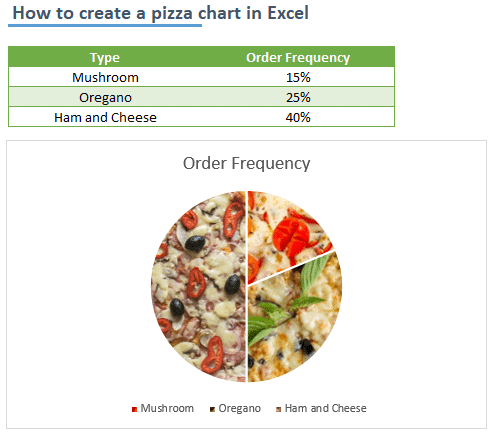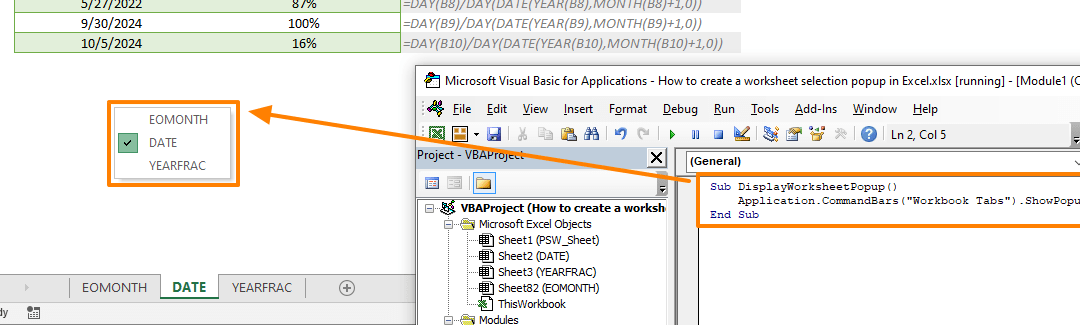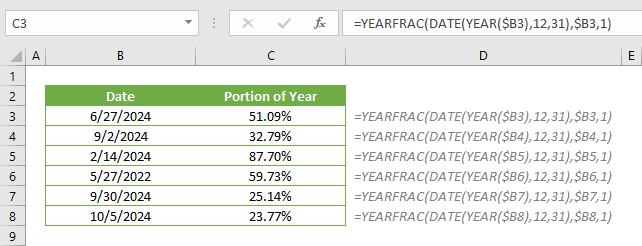Select Page## How to cut and paste transpose in Excel

A common problem when transposing data in Excel is that data is populated in their relative cells. This means that you may occasionally see messed up references when using the Paste function with transpose. In this article, we are going to show you how to cut and...## How to find the first non-blank cell in a range in Excel

If you frequently analyze data in Excel, you may come across empty cells before the actual values. Instead of removing these cells, you can get the position or even the value of the first non-blank cell with formulas. In this article, we are going to show you how to...## How to count the number of cells greater than the average in Excel

In this article, we are going to show you how to count the number of cells greater than the average in Excel. Download Workbook Formula =COUNTIFS(<numbers range>,”>“&AVERAGE(<numbers range>)) <numbers range>: The reference from the range of...## How to count the number of cells less than the average in Excel

In this article, we are going to show you how to count the number of cells that is less than the average of that data set in Excel. Download Workbook Formula =COUNTIFS(<numbers range>,”<“&AVERAGE(<numbers range>)) <numbers range>: The...## How to create a pizza chart in Excel

Of course, there isn’t a chart type called a pizza chart in Excel. On the other hand, Excel has a support for inserting images into chart elements. With help of this cool feature, you can give your charts a fancy look. In this article, we are going to show you how to...## How to create a worksheet selection popup in Excel

If your work requires navigating between worksheets a lot, activating those sheets can quickly become a hassle. Some VBA tips we’re going to cover here can help you to create a popup list of worksheets where you want them, and save yourself from the default...## How to calculate the percent of completed month

Have you ever wanted to track what percent of the month is completed? In this article, we are going to show you how to calculate the percent of completed month in Excel. If you want to calculate the percent of completed year instead, follow the link: How to calculate...## How to calculate the percent of completed year

A percentage value is a great way to express a proportion’s size in whole. You may want to calculate and see what percent of the year is completed. In this article, we are going to show you how to calculate the percent of completed year in Excel. Download Workbook...